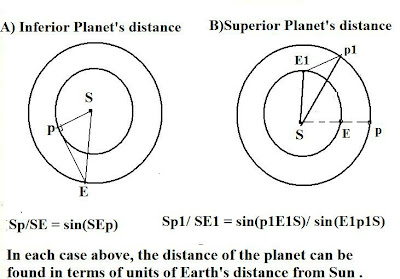## Tuesday, April 17, 2018

### Selected Questions- Answers From All Experts Astronomy Forum (Relative Distances of Superior, Inferior Planets)

Question: Can you explain how one can find the relative distance to an inferior or superior planet, i.e. in the solar system?

Answer:  Let's first define geometrically the inferior and superior planet based on the diagram shown below:As seen from the diagrams, an inferior planet defines one which is interior to Earth's orbit, relative to the Sun. A superior planet defines one which is exterior to the Earth's orbit.

While  it's easy to apply Kepler's 3rd law to obtain the basic dimensions of an orbit, namely the semi-major axis (a)  of the orbit - or the mean distance from the Sun, things become somewhat more difficult when we seek to find the distance say, of the Earth to the planet.

So, we consider two cases:

(A) Inferior planet (see the diagram A)

(B) Superior planet (Diagram B)

Case (A):

The maximum elongation occurs when the planet's geocentric radius vector (pE in diagram A) is perpendicular to the planet's heliocentric radius vector, pS. Then,  by a careful measurement of the angle SEp, say over a series of nights around maximum elongation, one can obtain a value for the angle of maximum elongation. At such time the angle SEp is right-angled hence:

Sp/ SE = sin(SEp)

The quantity Sp/SE is therefore the distance of the planet from the Sun in terms of Earth units (or AU, astronomical units, where 1 AU = 1.5 x 1011  m). Let Sp = R and SE = a(E) the semi-major axis for Earth's orbit, then:

Sp = R = sin(SEp) [a(E)]

Example:

If the angle SEp = 60 deg, find the planet's distance from the Sun.

Then: sin(SEp)= sin (60) = Ö3 /2 = 0.866

So: R = 0.866[a(E)]= 0.866 AU

Case (B):

Here, we let the planet p be in opposition at some given time with the Earth and Sun (e.g. showing the alignment S-E-p in diagram (B). As we know, with opposition, the elongation is a straight angle or 180 degrees. Then after t days have elapsed the Earth's radius vector SE has moved ahead of the planet's as shown in comparing SE1 to Sp1. As can be seen, this reduces the angle of elongation from 180 degrees at opposition to angle SE1p1. This is then measured.

Now, over t days, the angle ESp will have increased from 0 (at opposition) to a value Θ given by:

Θ = [n - n(p)] t

where n, n(p) are the mean daily motions of the Earth and the planet, respectively. Using relations for the periods (from the previous answer) we may write:

Θ = 360 (1/P - 1/P') t

where P and P' are the sidereal periods for the Earth and the planet, respectively.  Then, it follows by the synodic/ sidereal relations seen in previous answer.

Θ = 360 (1/S)

So, since t and S are both known, Θ can be obtained - that is, angle E1Sp1 is calculated. Hence, angle E1p1S can be found from:

angle E1p1S = 180 - angle SE1p1 - angle E1Sp1

From plane trigonometry we then obtain:

sin(p1E1S)/ Sp1 = sin(E1p1S)/SE1

or:

Sp1/ SE1 = sin(p1E1S)/ sin(E1p1S)

Again, giving the distance from the Sun in terms of Earth's distance unit.

To fix ideas here is a further  example problem:

Estimate the distance of Venus from the Sun at its most recent maximum elongation, if the angle of maximum  elongation was 46 degrees.

Solution:

The diagram is shown below with the angle of maximum elongation.We know, from the geometry:

SV/ SE = sin (SEV)

Then Venus' distance is:

SV = (sin(SEV)) SE

where: angle SEV = 46 deg and SE = a(E) = 1 AU

then:

SV = sin (46) 1 AU = (0.719) 1 AU = 0.719 AU